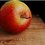# Proof that a pyramid of any base shape's volume is $\frac { 1 }{ 3 } Ah$ <-- yes “Ah”

My History exam just passed (yay) so this is just a small post to (celebrate?). Not that I posted a lot of notes. This is my first one.

Proof that a pyramid of any base shape's volume is $\frac { 1 }{ 3 } Ah$hi

In order to proof this, we are going to integrate the areas of the cross-section of the solid. Some of you would have already seen this:

$\int _{ b }^{ a }{ A(x)dx }$

Or

$\int _{ b }^{ a }{ A(y)dy }$

Where $A(x)$ is the function for the areas of the cross-section of the solid.

$!$

$\textbf{Finding A(x):}$

First consider this image:hi

Both triangles are right-angle triangles.

$(h)$ is the height of the solid and $(z)$ is just a constant such that ${ z }^{ 2 }\alpha A$

$(x)$ and $(y)$ are variables which would be used later to define $A(x)$

Since (${ z }^{ 2 }\alpha A$), we can express $A$ as $A=k{z}^{2}$

Where $(k)$ is just another constant. Keep in mind what this constant means, as we would be using it later.

Now, from the image above, it can be seen that both triangles are similar. So, finding the equation of $(y)$ with respect to variable $(x)$ is: $\frac { z }{ h } =\frac { y }{ x }$$y=\frac { xz }{ h }$

And therefore, $A(x)=k{y}^{2}=\frac{k{z}^{2}{x}^{2}}{{h}^{2}}$ The $(k)$ constant is used again to find the equation of the area of the cross-section of the object with respect to variable $(x)$.

$!$ $\textbf{Getting the volume:}$

The volume of the object is: $\int _{ b }^{ a }{ A(x)dx }$$\int _{ 0 }^{ h }{ \frac { k{ z }^{ 2 }{ x }^{ 2 } }{ { h }^{ 2 } } } =\frac { 1 }{ 3 } k{ z }^{ 2 }h$Recall that ($A=k{ z }^{ 2 }$). This makes $\frac { 1 }{ 3 } k{ z }^{ 2 }h=\boxed{\frac { 1 }{ 3 } Ah}$ Since the volume is based on the area of the cross section, the point at the top of the pyramid can literally be anywhere and this everybody-already-knows formula would still work. Thus, the Ah formula has been proved. Hope you liked this note.

$!$$!$

$\textbf{Additional: Volume of Sphere}$

There are 2 ways of doing this. One, is from the method I showed above, which… can be kind of boring….

The second way is a little more interesting.

You can visualise the sphere as made up of infinitely many pyramids. Whose height is the radius of the circle and their collective base area would be the surface area of the sphere. With…more…integration…you…could…find...the…surface…area…of…the…sphere. Which is boring. But we all know it's ($4\pi{r}^{2}$)

So, the volume of the Sphere would be:$\frac { 1 }{ 3 } Ah=\frac { 1 }{ 3 } (4\pi { r }^{ 2 })(r)=\boxed{\frac { 4 }{ 3 } \pi { r }^{ 3 }}$

Ok. This post isn't short.Note by Julian Poon
5 years, 4 months ago

This discussion board is a place to discuss our Daily Challenges and the math and science related to those challenges. Explanations are more than just a solution — they should explain the steps and thinking strategies that you used to obtain the solution. Comments should further the discussion of math and science.

When posting on Brilliant:

• Use the emojis to react to an explanation, whether you're congratulating a job well done , or just really confused .
• Ask specific questions about the challenge or the steps in somebody's explanation. Well-posed questions can add a lot to the discussion, but posting "I don't understand!" doesn't help anyone.
• Try to contribute something new to the discussion, whether it is an extension, generalization or other idea related to the challenge.

MarkdownAppears as
*italics* or _italics_ italics
**bold** or __bold__ bold
- bulleted- list
• bulleted
• list
1. numbered2. list
1. numbered
2. list
Note: you must add a full line of space before and after lists for them to show up correctly
paragraph 1paragraph 2

paragraph 1

paragraph 2

[example link](https://brilliant.org)example link
> This is a quote
This is a quote
    # I indented these lines
# 4 spaces, and now they show
# up as a code block.

print "hello world"
# I indented these lines
# 4 spaces, and now they show
# up as a code block.

print "hello world"
MathAppears as
Remember to wrap math in $$ ... $$ or $ ... $ to ensure proper formatting.
2 \times 3 $2 \times 3$
2^{34} $2^{34}$
a_{i-1} $a_{i-1}$
\frac{2}{3} $\frac{2}{3}$
\sqrt{2} $\sqrt{2}$
\sum_{i=1}^3 $\sum_{i=1}^3$
\sin \theta $\sin \theta$
\boxed{123} $\boxed{123}$

Sort by:

Apple's first note :P Thanks though, I got to know something new :)

- 3 years, 9 months ago

@Julian Poon Can you add this to a suitable Wiki page? Let me know if you want me to create a skill for you to post to.

Staff - 5 years, 4 months ago

@Calvin Lin I added it to Volume - Pyramid

- 5 years, 4 months ago

Sorry that the instructions were not clear.

I meant for you to click on "Write a summary", and paste the above text. This way, you will be adding directly to that wiki page, and others can come along and help improve it (like by providing a simplier initial explanation).

Staff - 5 years, 4 months ago

oh ok. Tomorrow. Its mid-night here :D

- 5 years, 4 months ago

ok. but how do you do so?

- 5 years, 4 months ago

Holy shit the sphere part was awesome!

- 5 years, 4 months ago

Nice! Check out my post.

- 5 years, 4 months ago